# SSAS: Discipline, Accuracy, Attention to Details. Part 2 – OLAP Cube

Total: 0 Average: 0

## Introduction

In this article, I will continue describing my experience with Microsoft Analysis Services. In addition to the previous article, I would like to write about unconventional solutions that were implemented in the recent project. These solutions got me close with Microsoft Analysis Services, and allowed me to do things that seemed to me impossible before.

## Period Average Sum

A customer wanted a sum of average values for each element per period, as it is shown below:### Variants of Solution

#### 1. First variant:

For this variant, we need to create a hidden measurement [ELEM COPY]. For this, we need to create a copy of [ELEM] and assign False to the Visible property. Next, we need to select “New Calculated Member” in the “Calculations” section of the cube, as shown below: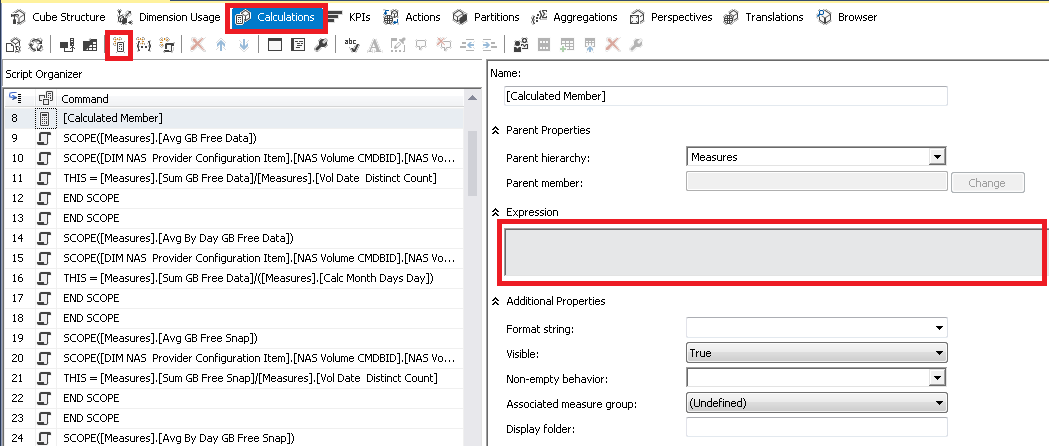And write the following in the Expression text box:

```iif (
not isleaf([ELEM].[ELEM SK].currentmember),
sum(EXISTING [ELEM COPY].[ELEM SK].currentmember.Children,[Measures].[FCT VAL]),
[Measures].[FCT VAL]
)```

Where [ELEM COPY].[ELEM SK] – is the key attribute of the hidden dimension.

[Measures].[FCT VAL] – is the AvarageOfChildren aggregation, i.e. when assembling the cube, the measurement of the AvarageOfChildren type was created and the cube calculated it automatically. Here is an example of how to create the calculation with the AvarageOfChildren aggregation: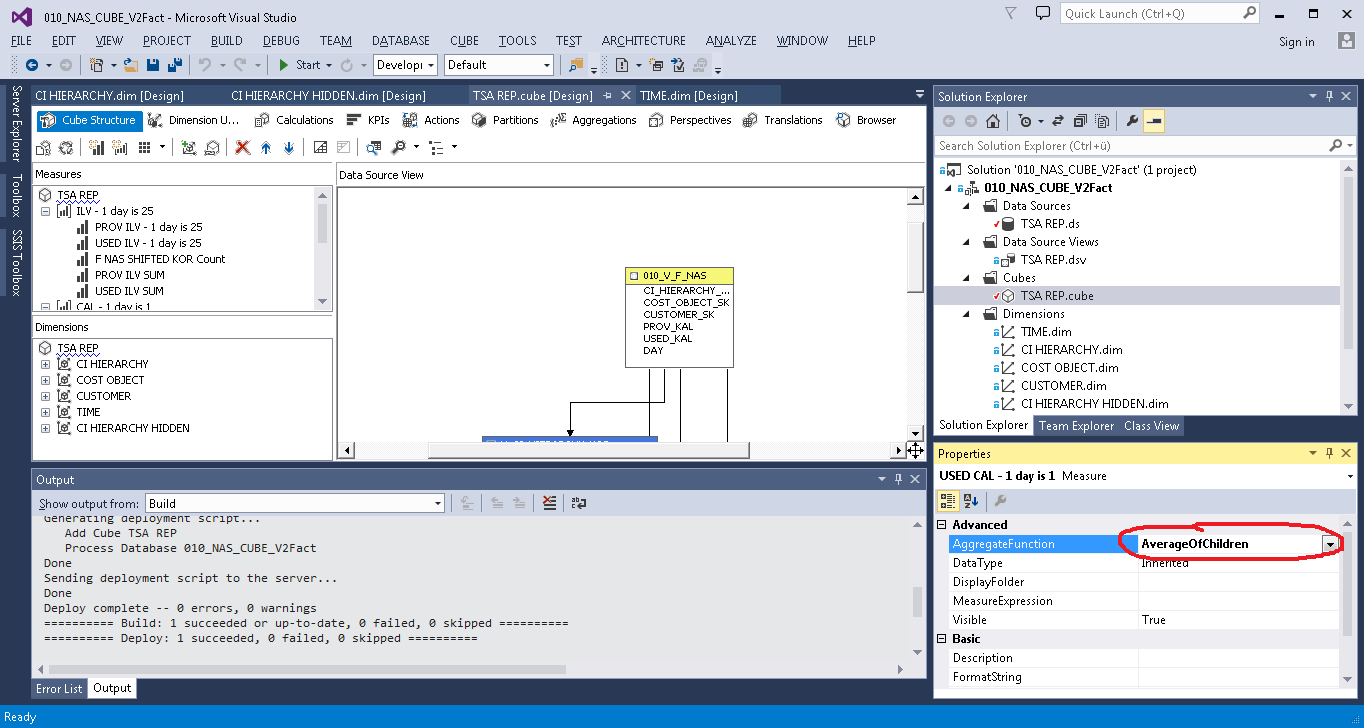#### 2. Solution with Scope

This solution was presented to me by a colleague of mine. In my opinion, it is more simple for understanding, though it is harder in terms of implementation.

What must be done:

We need to rewrite SELECT for the fact table. Instead of:

```SELECT [DATE]
,ID_CO
,ID_CUST
,ID_SYS
,ID_VOL
,ID_QUAL
,GB_used_DATA
FROM [000_REP].NAS_FACTS```

we need to write the following:

```SELECT [DATE]
,ID_CO
,ID_CUST
,ID_SYS
,ID_VOL
,ID_QUAL
,GB_used_DATA
,CONVERT(VARCHAR(10), [DATE], 120) + '|' + CAST(ID_VOL AS VARCHAR(MAX)) AS VolDate
,NULL AS Avg_GB_used_DATA
FROM [000_REP].NAS_FACTS```

Where GB_used_DATA is the fact we want to add to the cube. We need to make a non-standard behavior for the dimension with the ID_VOL key. As for the rest of dimensions with keys ID_CO, ID_CUST, ID_SYS and ID_QUAL, the behavior must remain standard, and in our task, everything must be simply summed up. Only for ID_VOL the period average must be calculated for each element, and the total for the ID_VOL elements must be summed up as well, the sum of average values for ID_VOL is expected as a result.

In the second query, we added 2 columns:

The first column uniquely determines the binding between the date and Id elements, for which the sum of average values must be calculated. A dimension with the DistinctCount aggregation function is added to the cube by this column. An example is shown below: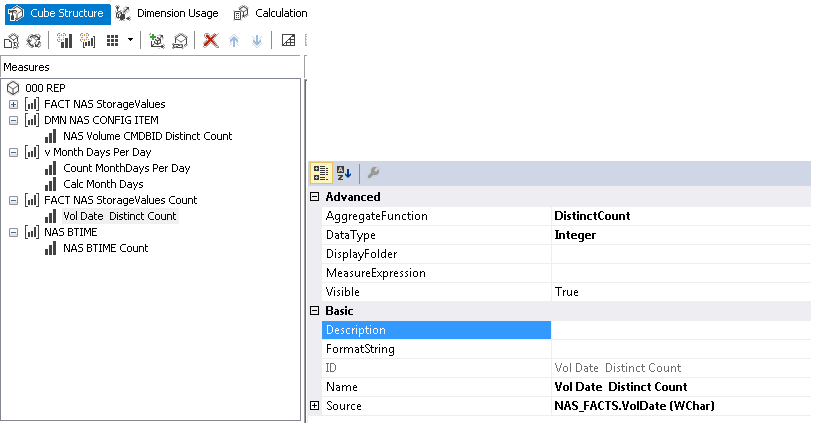The second column always stores the NULL value in all rows of the table. As for the second column, its name plays an important role – it is required for the creation of a dimension in the cube we can use for binding with the SCOPE function. It is also important that for this dimension, the Sum aggregation function is used. An example is shown below: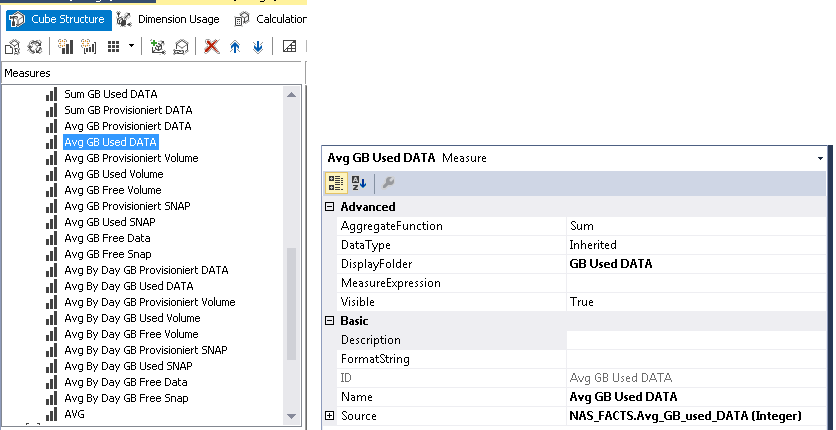Next, in the “Calculations” section of the cube, we need to select “Script View” and put the following code into the text box:

```SCOPE([Measures].[Avg GB Used DATA]);
SCOPE([ID_VOL Items].[ID VOL].[ID VOL].MEMBERS);
THIS = [Measures].[Sum GB Used DATA]/[Measures].[Vol Date  Distinct Count];
END SCOPE;
END SCOPE;```

where [ID_VOL Items] is a calculation with the ID_VOL key.

The image below shows the sequence of actions for this step:In this solution, we sum only the expression that is written in SCOPE, since without a formula in SCOPE, the NULL value that comes from the query to the database is stored there.

Both solutions gave identical result and calculated the average sum, as required.

## Statistical Average

After a while, the customer returned to the topic of calculation of average values. This time, he wanted a classical average instead of the average sum, i.e. he needed the same functionality as the AVERAGE function in Excel has. Since the customer constantly used the “Statistical Average” term, it became the title of this section.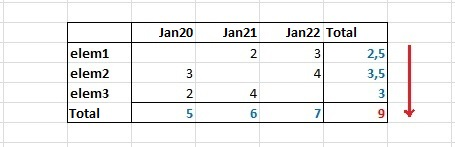We needed to calculate the average value for the whole range. An average for all elements for each day is divided by the number of days in the period. As a result, we get the average values for one element per period. The following solution was supposed:

```CREATE MEMBER CURRENTCUBE.[Measures].[Avg GB Used DATA (AvgAll Only valid days)]
as
[Measures].[Sum GB Used DATA]/[Measures].[Count VCMDB Only valid days],
VISIBLE = 1  ;

CREATE MEMBER CURRENTCUBE.[Measures].[Count VCMDB Only valid days]
as
Count(NonEmpty({crossjoin([DIM Business Time HD].[DAY].currentmember.Children,[DIM NAS  Provider Configuration Item HD].[NAS Volume CMDBID].currentmember.Children)}, [Measures].[Sum GB Free Data] )),
VISIBLE = 1  ;```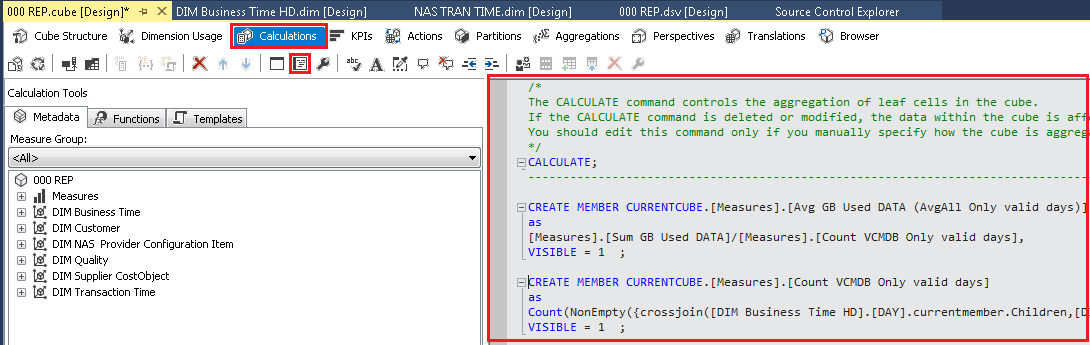In this solution, only the day where the element values were present were used. Also, we used a trick with a hidden dimension (these are dimensions [DIM Business Time HD].[DAY] and [DIM NAS Provider Configuration Item HD].[NAS Volume CMDBID]). We got the number of days with the values with help of crossjoin.

If we need to get the average for all values for all days, where the absence of values for a day is set equal to zero, I used the following expression:

```CREATE MEMBER CURRENTCUBE.[Measures].[Count VCMDB All days]
as
[Measures].[NAS Volume CMDBID Distinct Count] * [Measures].[NAS BTIME Count],
VISIBLE = 1  ;
CREATE MEMBER CURRENTCUBE.[Measures].[Count VCMDB All days]
as
[Measures].[NAS Volume CMDBID Distinct Count] * [Measures].[NAS BTIME Count],
VISIBLE = 1  ;```

where [Measures].[NAS Volume CMDBID Distinct Count] and [Measures].[NAS BTIME Count] are measures of the cube, that are built by the tables for dimensions (temporary dimension and element dimension):## One more useful function

During my work with the cube, it was required that the calculation of values must be changed depending on the hierarchy level. That is, if the days were selected, we would see the average per period; if months were selected, we would see the sum. It was implemented with help of the level function:

```CREATE MEMBER CURRENTCUBE.[Measures].[ML]
as
case
when [DIM Business Time].[HIERARCHY CAL].currentmember.level is [DIM Business Time].[HIERARCHY CAL].[YEAR] then 3
when [DIM Business Time].[HIERARCHY CAL].currentmember.level is [DIM Business Time].[HIERARCHY CAL].[PERIOD KAL] then 2
when [DIM Business Time].[HIERARCHY CAL].currentmember.level is [DIM Business Time].[HIERARCHY CAL].[DAY] then 1
else 4
end,
VISIBLE = 1;```

## Conclusion

Frankly speaking, when I saw the requirements to the calculation of average values, I was dazed and confused. I was dazed because sometimes the average values were either greater than the rest of values in the selected range (as in the example with the average sum) or less. I did not describe the case with less since didn’t quite understand it – the customer wanted a value for each day to be divided by the number of days in a month. I was confused because I had to implement this requirement somehow.

My attempts to cope with the task with help of the standard AvarageOfChildren (that sums all figures for a day, than adds all sums and divides by the number of days in the period) have failed. The customer insisted on correspondence to his view of the world. All our solutions fell under the firm and rigorous testing. The question of confidence between us and the customer has been removed at the early steps of our interaction. He was constantly looking for nasty tricks from us, and the solution has turned into something weird and monstrous instead of being simple and user-friendly. But every cloud has a silver lining. Owing to such requirements, we had to go deep into the work of the OLAP cube and learned to work with the random aggregation. This experience turned out really useful for me. So, I decided to share it with you.#### Alexander Korolev

Alexander graduated in computer science, and later in accounting. He has been working as a software developer since 2000: started as a C ++ developer, worked with .NET for a long time, parallelly working with databases (DWH, Business Intelligence, OLAP). Alexander has experience with Java technologies.